# NCERT Solutions for Class 9 Science Chapter 12 – Sound

NCERT Solutions for Class 9 Science Chapter 12 – Sound, contains solutions to various questions in Exercises for Chapter 12. Sound Class 9 NCERT Solutions have been explained in a simple and easy to understand manner. We are providing all subjects which can be accessed by clicking here.

## Download NCERT Solutions for Class 9 Science Chapter 12 – Sound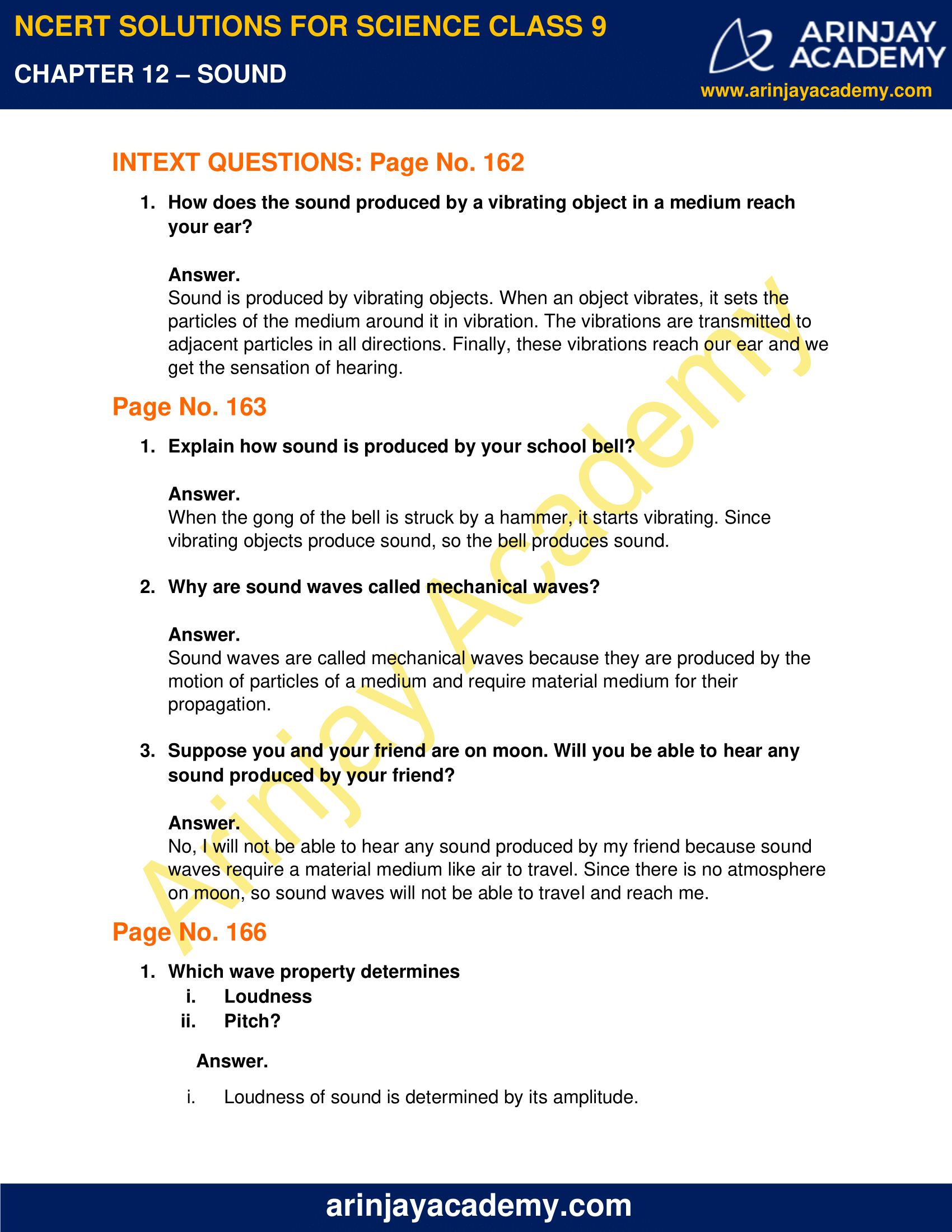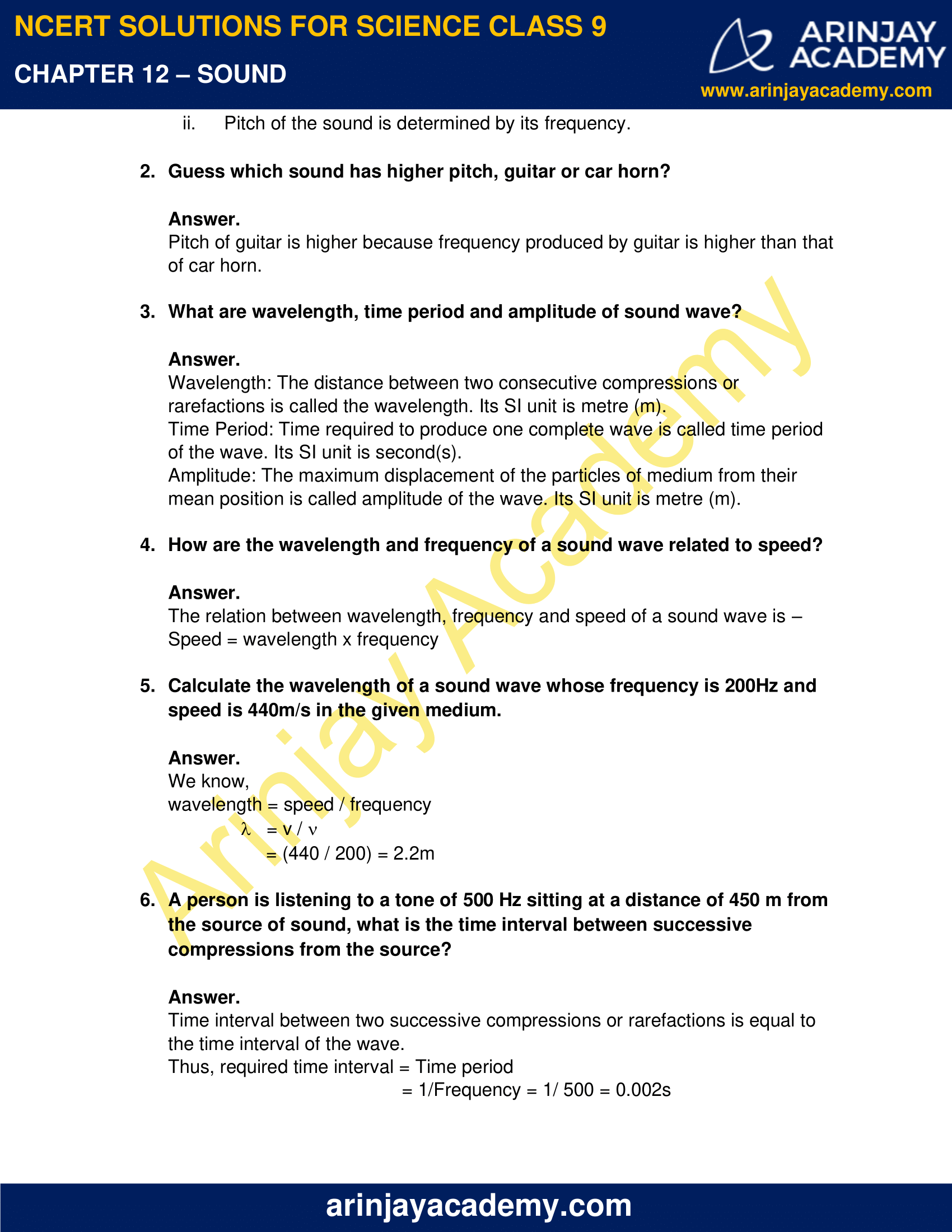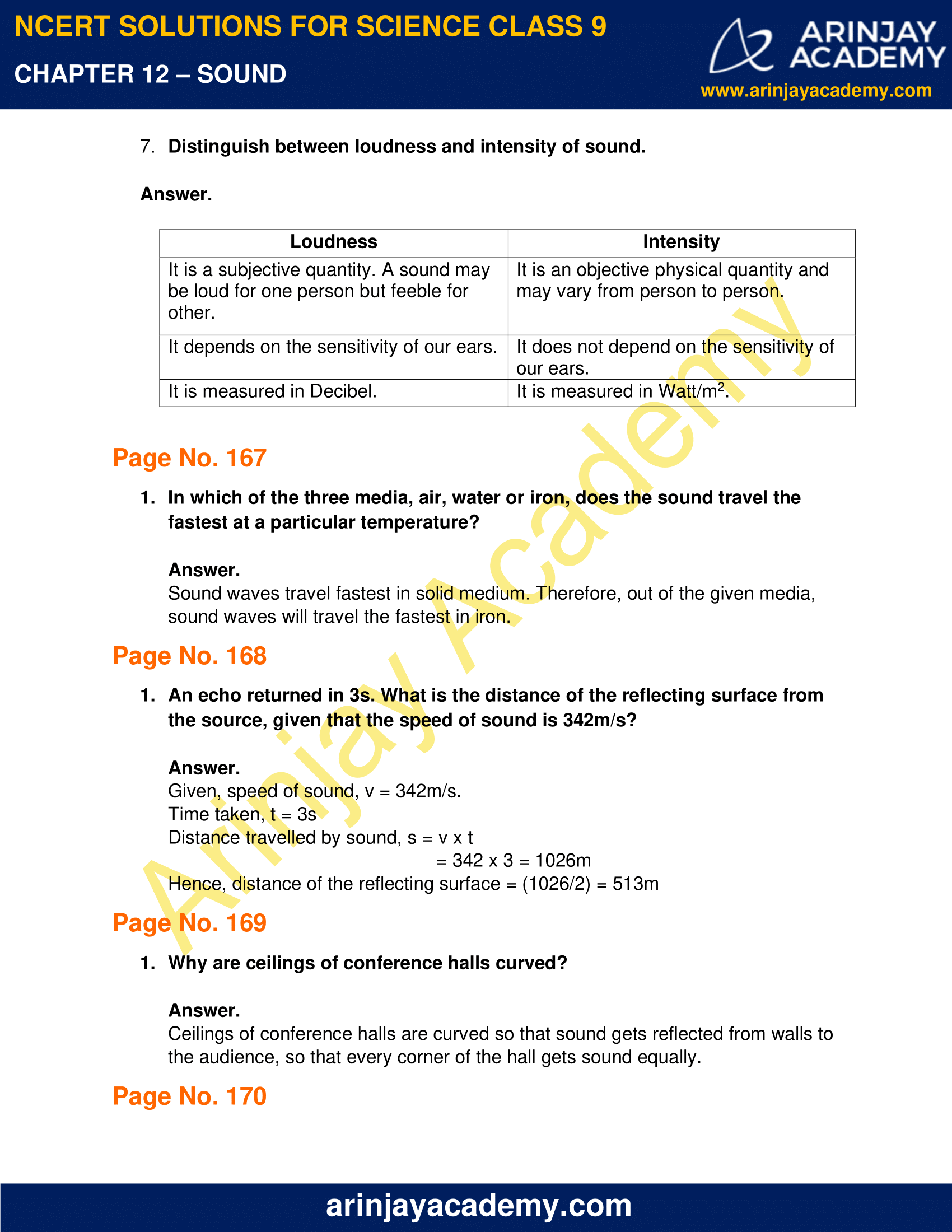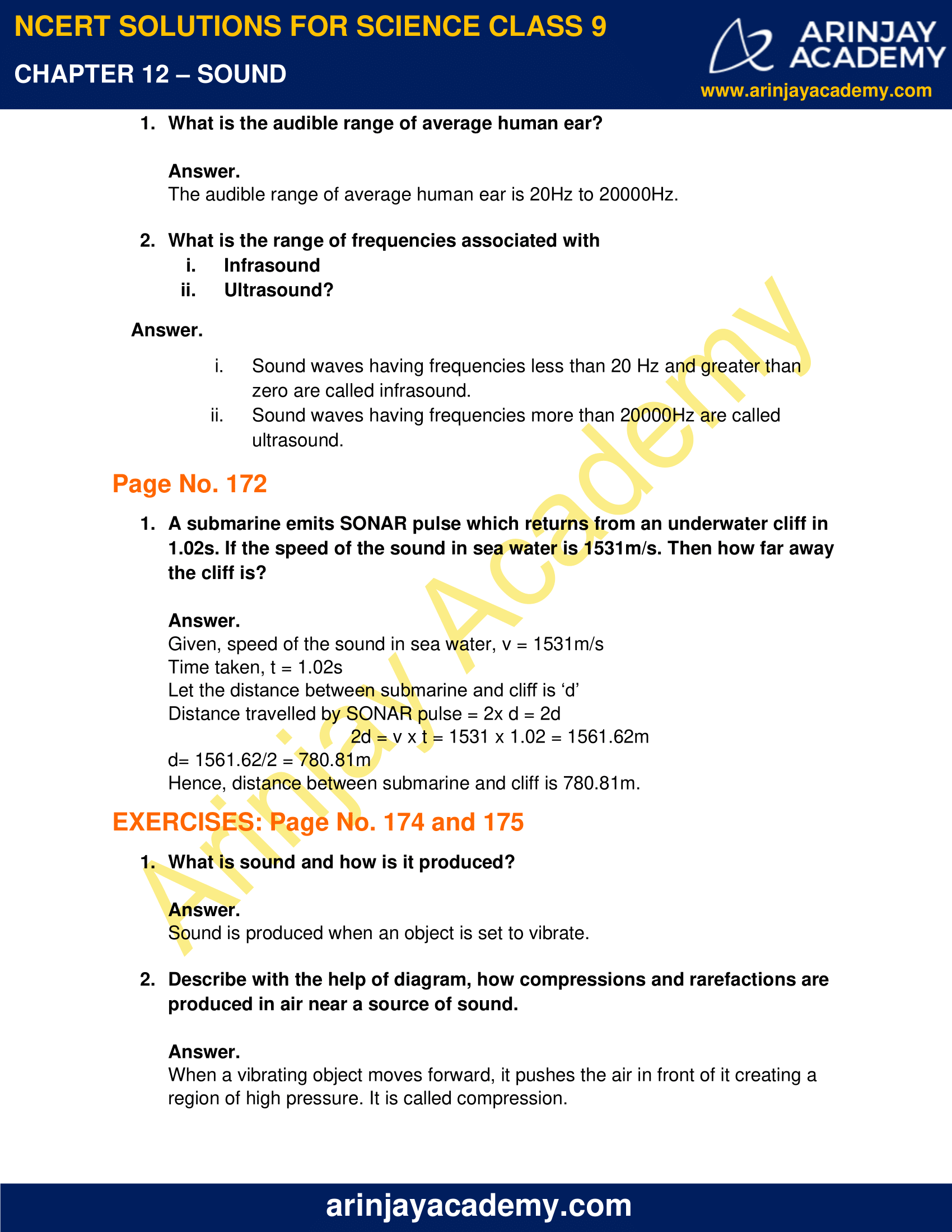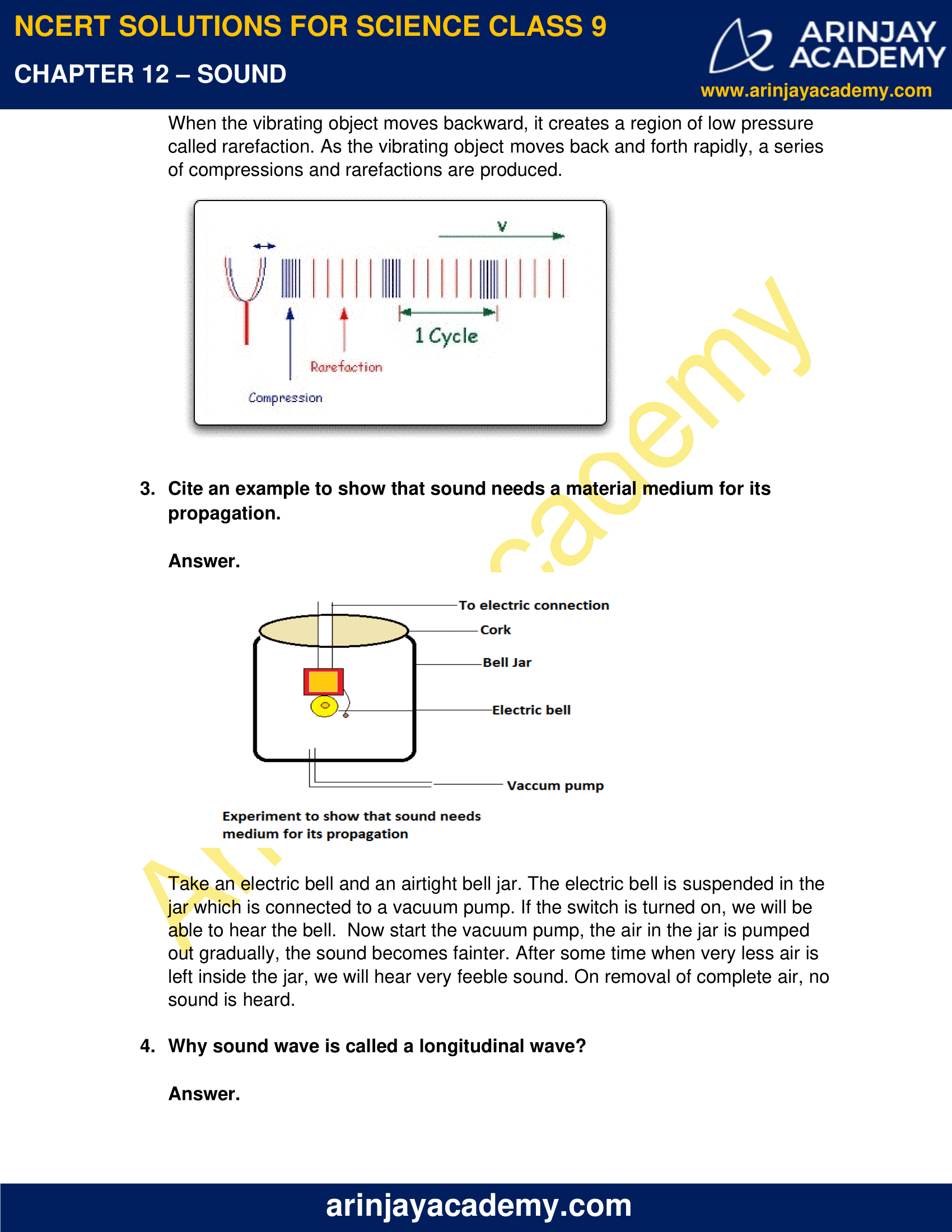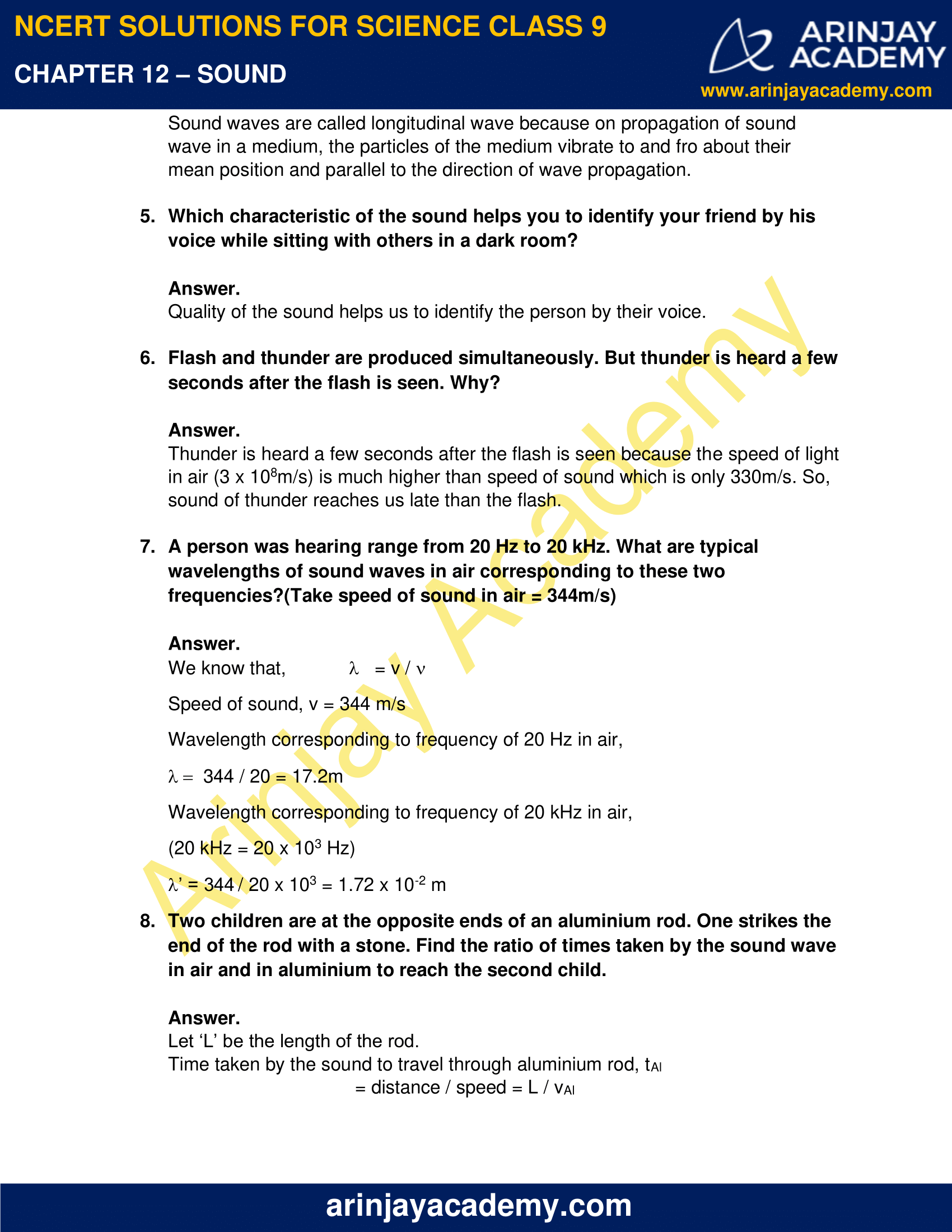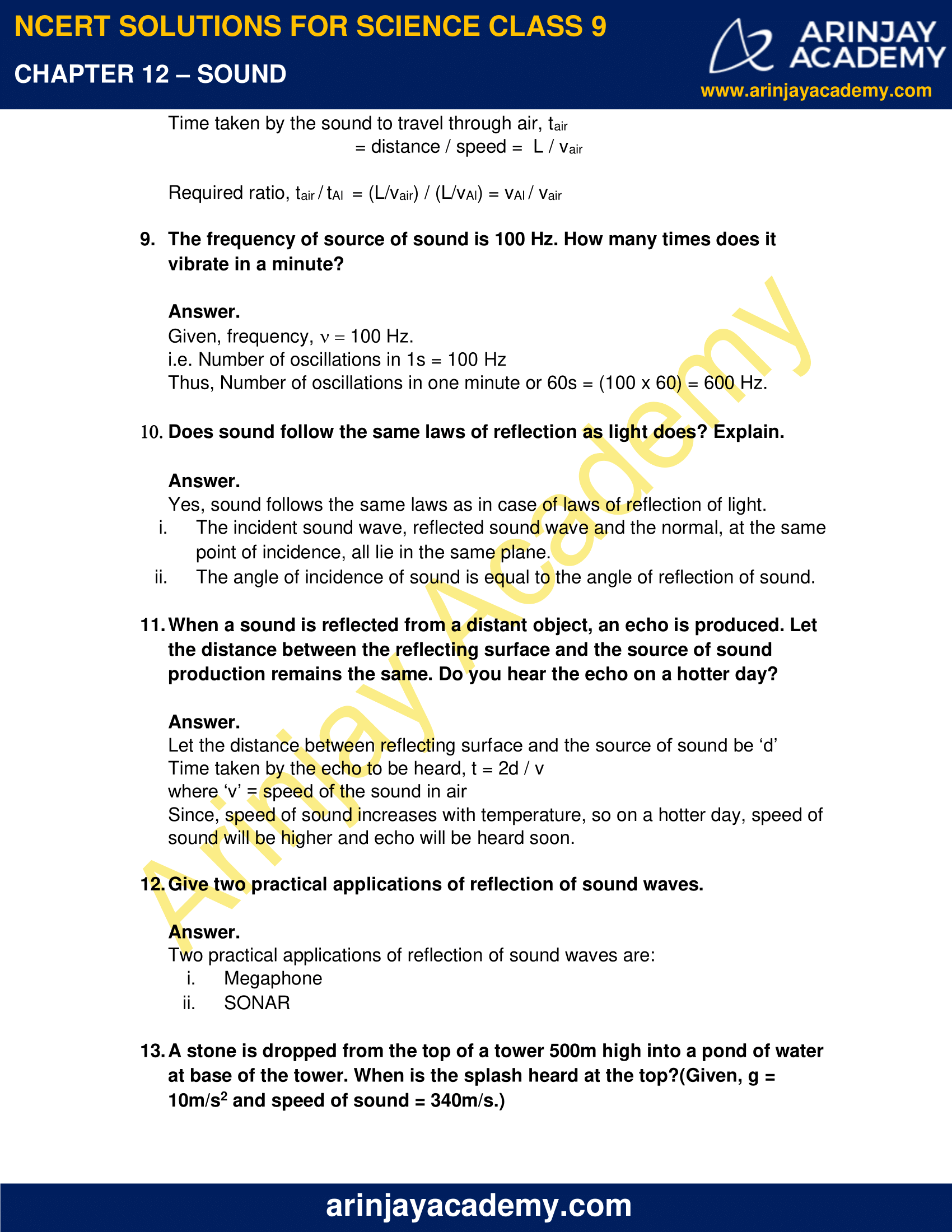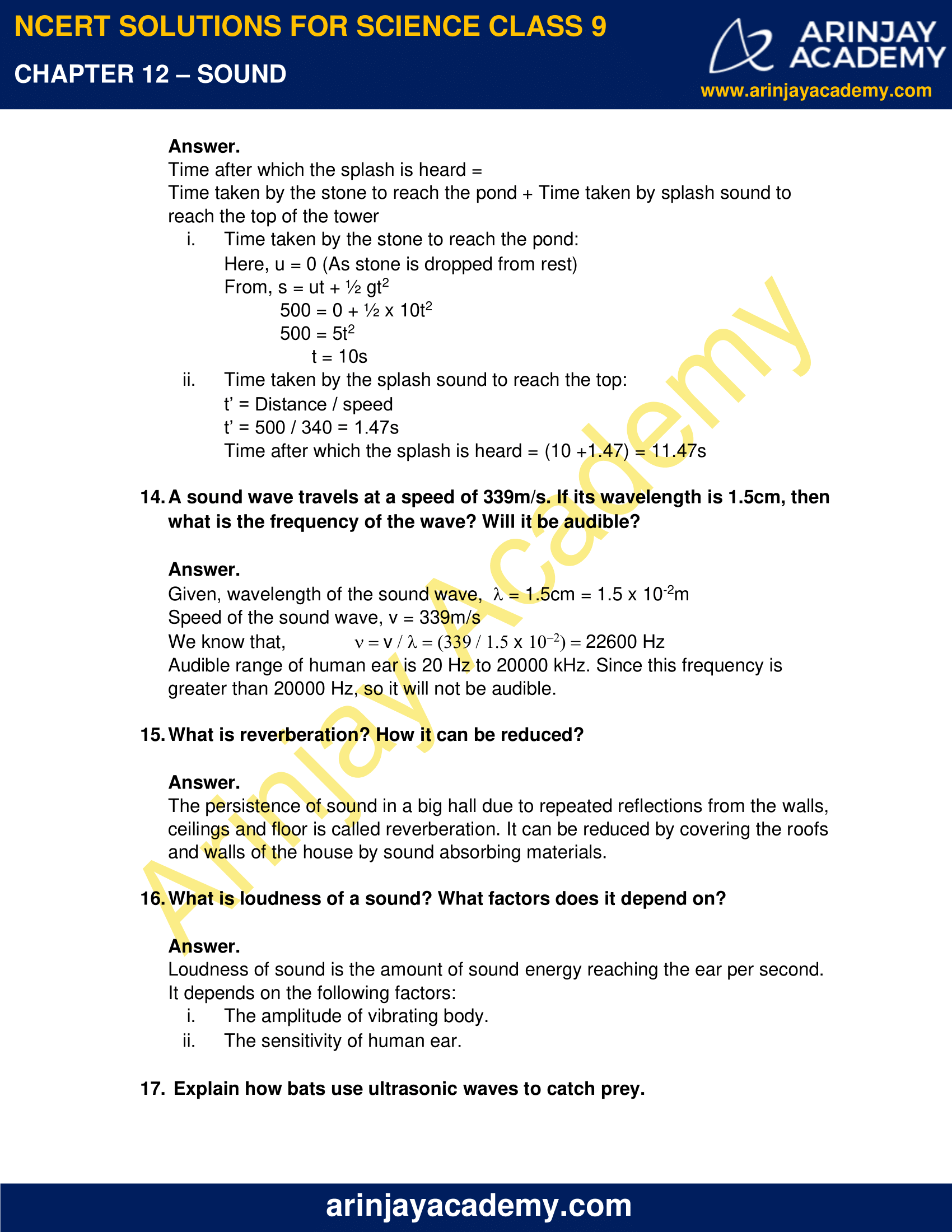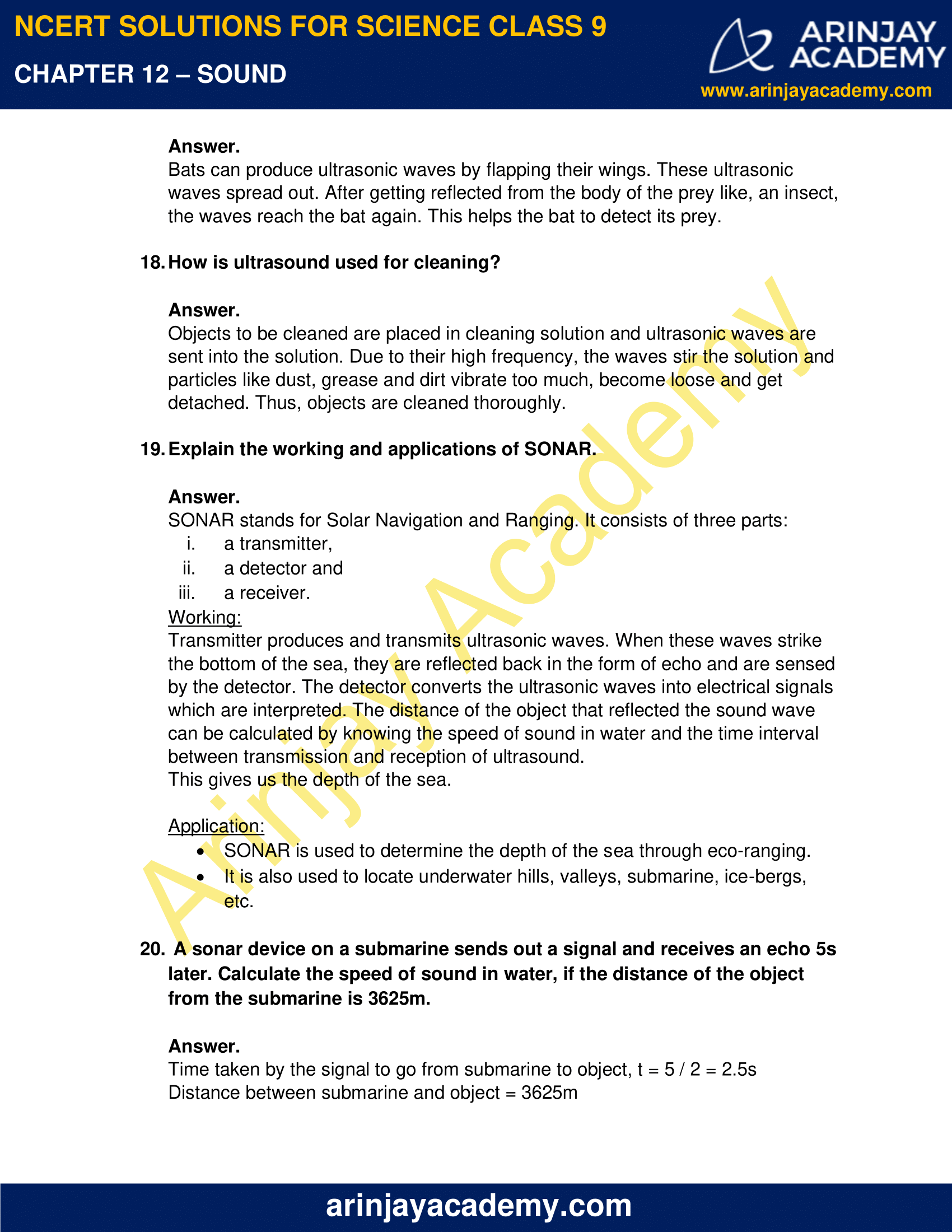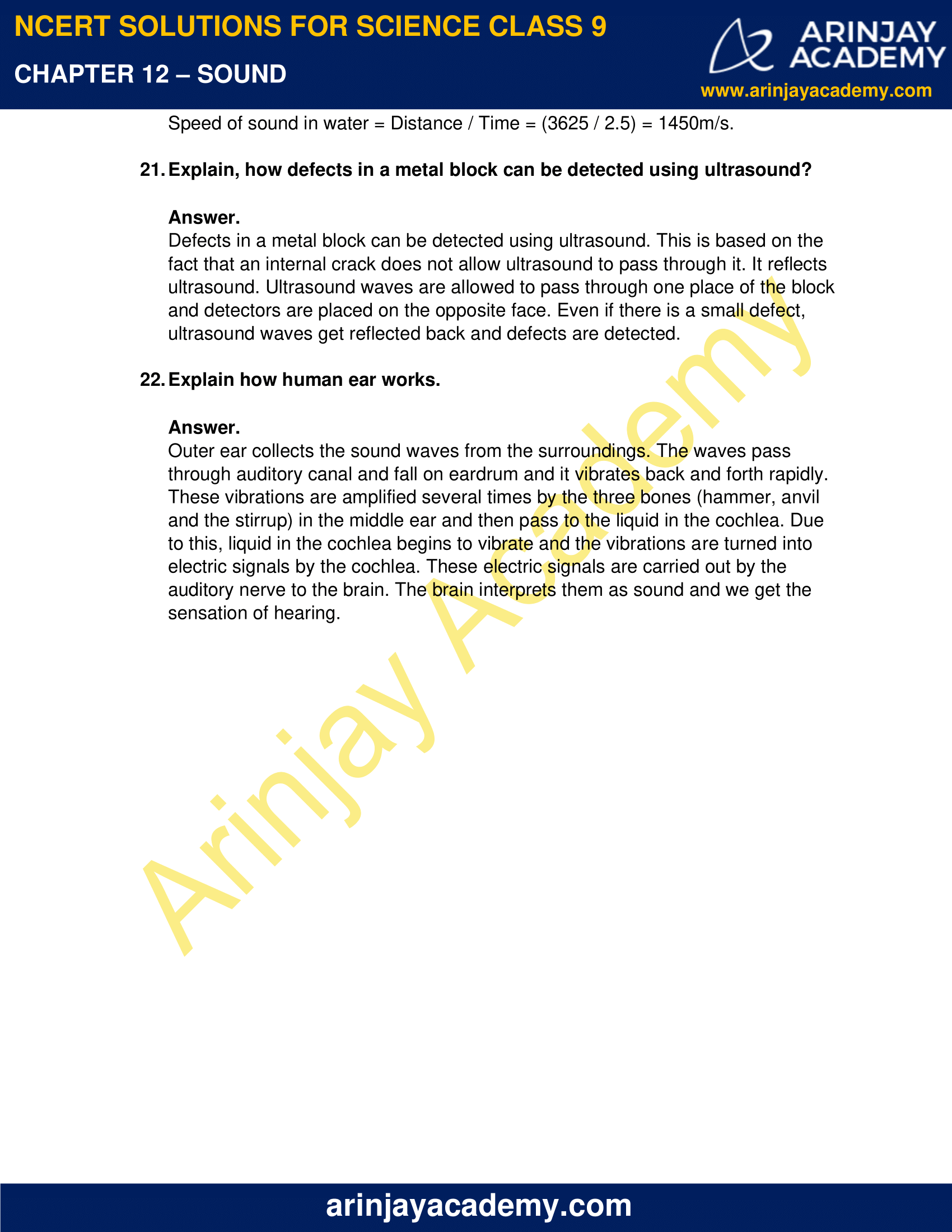### NCERT Solutions for Class 9 Science Chapter 12 – Sound – NCERT Exercises

NCERT Solutions for Class 9 Science Chapter 12 – Sound, Exercises includes all in text and exercise solved questions which helps you to understand the topic covered in Chapter 12 Sound, in a better manner to help you to score good marks in your examinations.

### NCERT Solutions for Class 9 Science Chapter 12 – Sound – Intext Questions – Page No. 162

1. How does the sound produced by a vibrating object in a medium reach your ear?

Answer.

Sound is produced by vibrating objects. When an object vibrates, it sets the particles of the medium around it in vibration. The vibrations are transmitted to adjacent particles in all directions. Finally, these vibrations reach our ear and we get the sensation of hearing.

### Page No. 163

1. Explain how sound is produced by your school bell?

Answer.

When the gong of the bell is struck by a hammer, it starts vibrating. Since vibrating objects produce sound, so the bell produces sound.

2. Why are sound waves called mechanical waves?

Answer.

Sound waves are called mechanical waves because they are produced by the motion of particles of a medium and require material medium for their propagation.

3. Suppose you and your friend are on moon. Will you be able to hear any sound produced by your friend?

Answer.

No, I will not be able to hear any sound produced by my friend because sound waves require a material medium like air to travel. Since there is no atmosphere on moon, so sound waves will not be able to travel and reach me.

### NCERT Solutions for Class 9 Science Chapter 12 – Sound – Intext Questions – Page No. 166

1. Which wave property determines

i. Loudness
ii. Pitch?

Answer.

i. Loudness of sound is determined by its amplitude.
ii. Pitch of the sound is determined by its frequency.

2. Guess which sound has higher pitch, guitar or car horn?

Answer.

Pitch of guitar is higher because frequency produced by guitar is higher than that of car horn.

3. What are wavelength, time period and amplitude of sound wave?

Answer.

Wavelength: The distance between two consecutive compressions or rarefactions is called the wavelength. Its SI unit is metre (m).

Time Period: Time required to produce one complete wave is called time period of the wave. Its SI unit is second(s).

Amplitude: The maximum displacement of the particles of medium from their mean position is called amplitude of the wave. Its SI unit is metre (m).

4. How are the wavelength and frequency of a sound wave related to speed?

Answer.

The relation between wavelength, frequency and speed of a sound wave is –

Speed = wavelength x frequency

5. Calculate the wavelength of a sound wave whose frequency is 200Hz and speed is 440m/s in the given medium.

Answer.

We know,

wavelength = speed / frequency

l   = v / n

= (440 / 200) = 2.2m

6. A person is listening to a tone of 500 Hz sitting at a distance of 450 m from the source of sound, what is the time interval between successive compressions from the source?

Answer.

Time interval between two successive compressions or rarefactions is equal to the time interval of the wave.

Thus, required time interval = Time period

= 1/Frequency = 1/ 500 = 0.002s

7. Distinguish between loudness and intensity of sound.

Answer.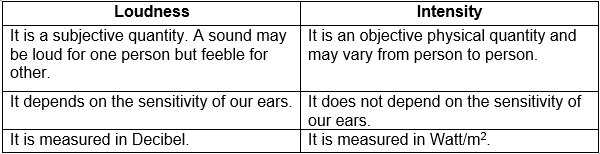### NCERT Solutions for Class 9 Science Chapter 12 – Sound – Intext Questions – Page No. 167

1. In which of the three media, air, water or iron, does the sound travel the fastest at a particular temperature?

Answer.

Sound waves travel fastest in solid medium. Therefore, out of the given media, sound waves will travel the fastest in iron.

### Page No. 168

1. An echo returned in 3s. What is the distance of the reflecting surface from the source, given that the speed of sound is 342m/s?

Answer.

Given, speed of sound, v = 342m/s.

Time taken, t = 3s

Distance travelled by sound, s = v x t

= 342 x 3 = 1026m

Hence, distance of the reflecting surface = (1026/2) = 513m

### Page No. 169

1. Why are ceilings of conference halls curved?

Answer.

Ceilings of conference halls are curved so that sound gets reflected from walls to the audience, so that every corner of the hall gets sound equally.

### Page No. 170

1. What is the audible range of average human ear?

Answer.

The audible range of average human ear is 20Hz to 20000Hz.

2. What is the range of frequencies associated with

i. Infrasound
ii. Ultrasound?

Answer.

i. Sound waves having frequencies less than 20 Hz and greater than zero are called infrasound.
ii. Sound waves having frequencies more than 20000 Hz are called ultrasound.

### Page No. 172

1. A submarine emits SONAR pulse which returns from an underwater cliff in 1.02s. If the speed of the sound in sea water is 1531m/s. Then how far away the cliff is?

Answer.

Given, speed of the sound in sea water, v = 1531m/s

Time taken, t = 1.02s

Let the distance between submarine and cliff is ‘d’

Distance travelled by SONAR pulse = 2x d = 2d

2d = v x t = 1531 x 1.02 = 1561.62m

d= 1561.62/2 = 780.81m

Hence, distance between submarine and cliff is 780.81m.

### NCERT Solutions for Class 9 Science Chapter 12 – Sound – NCERT Exercise – Page No. 174 and 175

1. What is sound and how is it produced?

Answer.

Sound is produced when an object is set to vibrate.

2. Describe with the help of diagram, how compressions and rarefactions are produced in air near a source of sound.

Answer.

When a vibrating object moves forward, it pushes the air in front of it creating a region of high pressure. It is called compression.

When the vibrating object moves backward, it creates a region of low pressure called rarefaction. As the vibrating object moves back and forth rapidly, a series of compressions and rarefactions are produced.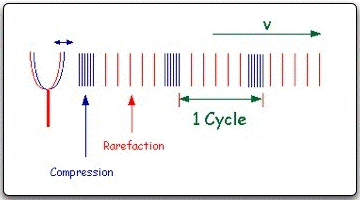3. Cite an example to show that sound needs a material medium for its propagation.

Answer.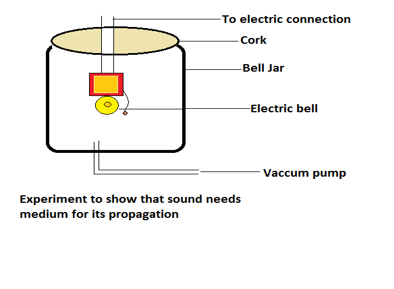Take an electric bell and an airtight bell jar. The electric bell is suspended in the jar which is connected to a vacuum pump. If the switch is turned on, we will be able to hear the bell.  Now start the vacuum pump, the air in the jar is pumped out gradually, the sound becomes fainter. After some time when very less air is left inside the jar, we will hear very feeble sound. On removal of complete air, no sound is heard.

4. Why sound wave is called a longitudinal wave?

Answer.

Sound waves are called longitudinal wave because on propagation of sound wave in a medium, the particles of the medium vibrate to and fro about their mean position and parallel to the direction of wave propagation.

5. Which characteristic of the sound helps you to identify your friend by his voice while sitting with others in a dark room?

Answer.

Quality of the sound helps us to identify the person by their voice.

6. Flash and thunder are produced simultaneously. But thunder is heard a few seconds after the flash is seen. Why?

Answer.

Thunder is heard a few seconds after the flash is seen because the speed of light in air (3 x 108m/s) is much higher than speed of sound which is only 330m/s. So, sound of thunder reaches us late than the flash.

7. A person was hearing range from 20 Hz to 20 kHz. What are typical wavelengths of sound waves in air corresponding to these two frequencies?(Take speed of sound in air = 344m/s)

Answer.

We know that, l = v / n

Speed of sound, v = 344 m/s

Wavelength corresponding to frequency of 20 Hz in air,

l =  344 / 20 = 17.2m

Wavelength corresponding to frequency of 20 kHz in air,

(20 kHz = 20 x 103 Hz)

l’ = 344 / 20 x 103 = 1.72 x 10-2 m

8. Two children are at the opposite ends of an aluminium rod. One strikes the end of the rod with a stone. Find the ratio of times taken by the sound wave in air and in aluminium to reach the second child.

Answer.

Let ‘L’ be the length of the rod.

Time taken by the sound to travel through aluminium rod, tAl

= distance / speed = L / vAl

Time taken by the sound to travel through air, tair

= distance / speed =  L / vair

Required ratio, tair / tAl  = (L/vair) / (L/vAl) = vAl / vair

9. The frequency of source of sound is 100 Hz. How many times does it vibrate in a minute?

Answer.

Given, frequency, n = 100 Hz.

i.e. Number of oscillations in 1s = 100 Hz

Thus, Number of oscillations in one minute or 60s = (100 x 60) = 600 Hz.

10. Does sound follow the same laws of reflection as light does? Explain.

Answer.

Yes, sound follows the same laws as in case of laws of reflection of light.

i. The incident sound wave, reflected sound wave and the normal, at the same point of incidence, all lie in the same plane.
ii. The angle of incidence of sound is equal to the angle of reflection of sound.

11. When a sound is reflected from a distant object, an echo is produced. Let the distance between the reflecting surface and the source of sound production remains the same. Do you hear the echo on a hotter day?

Answer.

Let the distance between reflecting surface and the source of sound be ‘d’

Time taken by the echo to be heard, t = 2d / v

where ‘v’ = speed of the sound in air

Since, speed of sound increases with temperature, so on a hotter day, speed of sound will be higher and echo will be heard soon.

12. Give two practical applications of reflection of sound waves.

Answer.

Two practical applications of reflection of sound waves are:

1. Megaphone
2. SONAR

13. A stone is dropped from the top of a tower 500m high into a pond of water at base of the tower. When is the splash heard at the top?(Given, g = 10m/s2 and speed of sound = 340m/s.)

Answer.

Time after which the splash is heard = Time taken by the stone to reach the pond + Time taken by splash sound to reach the top of the tower

i. Time taken by the stone to reach the pond:

Here, u = 0 (As stone is dropped from rest)

From, s = ut + ½ gt2

500 = 0 + ½ x 10t2

500 = 5t2

t = 10s

ii. Time taken by the splash sound to reach the top:

t’ = Distance / speed

t’ = 500 / 340 = 1.47s

Time after which the splash is heard = (10 +1.47) = 11.47s

14. A sound wave travels at a speed of 339m/s. If its wavelength is 1.5cm, then what is the frequency of the wave? Will it be audible?

Answer.

Given, wavelength of the sound wave,  l = 1.5cm = 1.5 x 10-2m

Speed of the sound wave, v = 339m/s

We know that,  n = v / l = (339 / 1.5 x 10-2) = 22600 Hz

Audible range of human ear is 20 Hz to 20000 kHz. Since this frequency is greater than 20000 Hz, so it will not be audible.

15. What is reverberation? How it can be reduced?

Answer.

The persistence of sound in a big hall due to repeated reflections from the walls, ceilings and floor is called reverberation. It can be reduced by covering the roofs and walls of the house by sound absorbing materials.

16. What is loudness of a sound? What factors does it depend on?

Answer.

Loudness of sound is the amount of sound energy reaching the ear per second.

It depends on the following factors:

i. The amplitude of vibrating body.
ii. The sensitivity of human ear.

17. Explain how bats use ultrasonic waves to catch prey.

Answer.

Bats can produce ultrasonic waves by flapping their wings. These ultrasonic waves spread out. After getting reflected from the body of the prey like, an insect, the waves reach the bat again. This helps the bat to detect its prey.

18. How is ultrasound used for cleaning?

Answer.

Objects to be cleaned are placed in cleaning solution and ultrasonic waves are sent into the solution. Due to their high frequency, the waves stir the solution and  particles like dust, grease and dirt vibrate too much, become loose and get detached. Thus, objects are cleaned thoroughly.

19. Explain the working and applications of SONAR.

Answer.

SONAR stands for Solar Navigation and Ranging. It consists of three parts:

i. a transmitter,
ii. a detector and
iii. a receiver.

Working:

Transmitter produces and transmits ultrasonic waves. When these waves strike the bottom of the sea, they are reflected back in the form of echo and are sensed by the detector. The detector converts the ultrasonic waves into electrical signals which are interpreted. The distance of the object that reflected the sound wave can be calculated by knowing the speed of sound in water and the time interval between transmission and reception of ultrasound.

This gives us the depth of the sea.

Application:

• SONAR is used to determine the depth of the sea through eco-ranging.
• It is also used to locate underwater hills, valleys, submarine, ice-bergs, etc.

20. A sonar device on a submarine sends out a signal and receives an echo 5s later. Calculate the speed of sound in water, if the distance of the object from the submarine is 3625m.

Answer.

Time taken by the signal to go from submarine to object, t = 5 / 2 = 2.5s

Distance between submarine and object = 3625m

Speed of sound in water = Distance / Time = (3625 / 2.5) = 1450m/s.

21. Explain, how defects in a metal block can be detected using ultrasound?

Answer.

Defects in a metal block can be detected using ultrasound. This is based on the fact that an internal crack does not allow ultrasound to pass through it. It reflects ultrasound. Ultrasound waves are allowed to pass through one place of the block and detectors are placed on the opposite face. Even if there is a small defect, ultrasound waves get reflected back and defects are detected.

22. Explain how human ear works.

Answer.

Outer ear collects the sound waves from the surroundings. The waves pass through auditory canal and fall on eardrum and it vibrates back and forth rapidly. These vibrations are amplified several times by the three bones (hammer, anvil and the stirrup) in the middle ear and then pass to the liquid in the cochlea. Due to this, liquid in the cochlea begins to vibrate and the vibrations are turned into electric signals by the cochlea. These electric signals are carried out by the auditory nerve to the brain. The brain interprets them as sound and we get the sensation of hearing.

### Topics Covered in Sound Class 9 Science

1. Production of Sound
2. Propagation of Sound
3. Reflection of Sound
4. Range of Hearing
5. Applications of Ultrasound
6. Structure of Human Ear